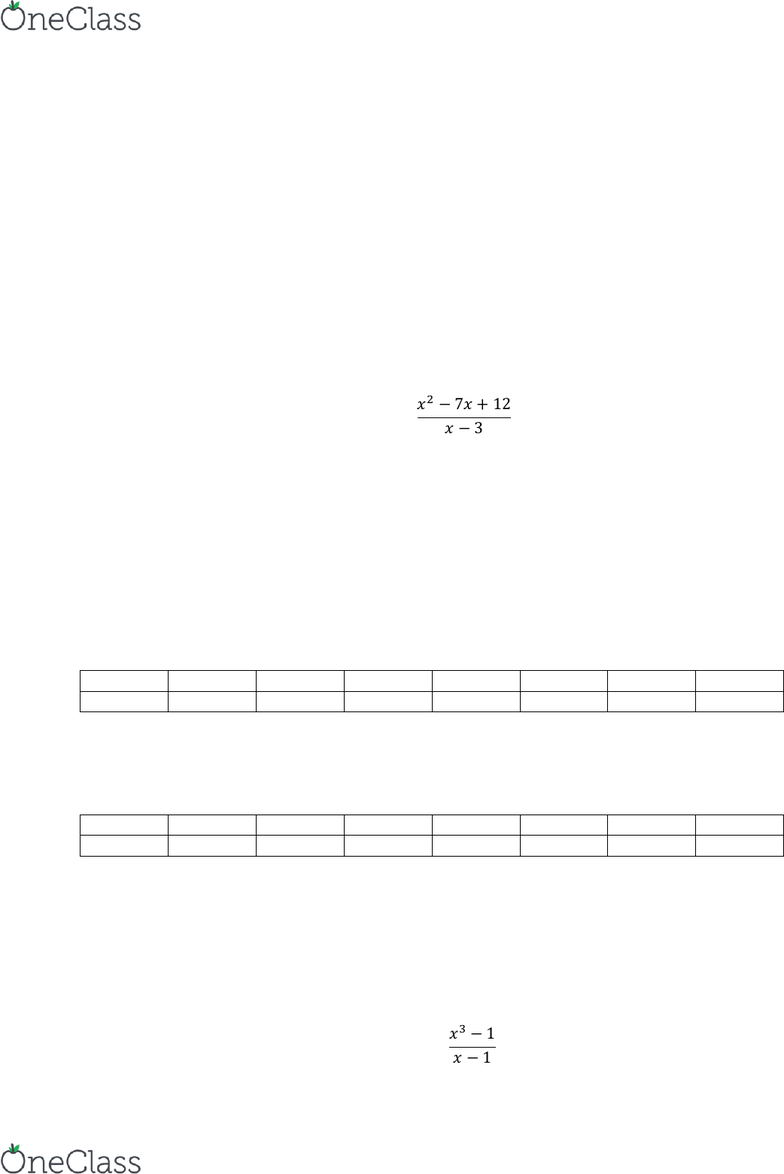# MATH 161 Lecture 2: Calculus 1- Limits: Table Method

34 views3 pages
School
Department
Course
Page:
of 3Calculus 1
Chapter 2, Section 2 Intro to Limits (cont.) and Their Properties
Prior to this, were learning how to solve limits as x approaches a number analytically with use of algebra,
but now we are going to look at how to solve a limit using a table method.
Dont worry it is not anything hard, it concludes of making a table and writing down close numbers to the
left and right of the limit and plugging those values to find values that draw close to the limit. This a pretty
simple method to follow.
Let’s look at an example which we can solve for the limit using the table method:
Ex.1:
  



First, we must find what make the f(x)= 0, which is 3 as x approaches 3 the whole function becomes 0, so
in our table when x is at 3, it will be undefined. The key note is that a limit is not at a value but a close to
that value. Now that know that the f(x)=0 at 3, we must find numbers to left of 3 (less than 3) like 2, and
values much closer like 2.9 and 2.99. Also find numeric values closest to 3 on the right side (greater than
3) as well in order to set up our table like 3.01, 3.1 and 4.
Step 1: Set up table with the following x-values.
x
2
2.9
2.99
3
3.01
3.1
4
F(x)
Step 2: Plug in the remaining values by plugging in the x-values into the equation above to find the limit.
x
2
2.9
2.99
3
3.01
3.1
4
F(x)
-2
-1.1
-1.01
DNE
-1.01
-1.1
-2
-As you can see as we get closer to 3 from the left and right, we are getting closer to -1 and that is our
limit.
See it was not that bad as long as you master the concept. Let try another to make sure you got the hang
of it.
Ex.2:
 

find more resources at oneclass.com
find more resources at oneclass.com
According to the following f(x), we need to find the value that makes the f(x) =0, which is 1 as x goes to 1.
We must choose numbers close to the 1 from the left and right. From the left, we can choose numbers
closest to 1 such as 0.9, 0.99 and 0.999. Also from the right, the numeric values closest to 1 such as 1.001,
1.01 and 1.1.
Step 1: Set up a table with the following values mentioned above.
x
0.9
0.99
0.999
1
1.001
1.01
1.1
f(x)
Step 2: Plug in the remaining values by plugging in the x-values into the equation above to find the limit.
x
0.9
0.99
0.999
1
1.001
1.01
1.1
f(x)
2.71
2.97
2.999
DNE
3.001
3.03
3.31
As we get closer to 1, we find out that we are getting closer to 3 from the left and right side. Therefore, 3
is our limit.
See that was not too bad! Let try another example just to make sure we have mastered the limit by using
the table method.
Ex.3:
 

 
According to the f(x), we need to find the numeric value that makes f(x) =0, which is numerical value, 0.
We need to find numbers from the left and right. From the left side of 0, we can use numerical values
such as -0.1,-0.01,-0.001. Also from the right side of 0, we can use the 0.001, 0.01 and 0.1.
Step 1: Set up a table with the following values mentioned above.
x
-0.1
-0.01
-0.001
0
0.001
0.01
0.1
f(x)
Step 2: Plug in the remaining values by plugging in the x-values into the equation above to find the limit.
x
-0.1
-0.01
-0.001
0
0.001
0.01
0.1
f(x)
1.95
1.995
1.9995
DNE
2.0005
2.005
2.05
As you get closer to the number 0, the values from the left and right side are getting closer from the
numerical value, 2. Therefore, our limit is 2.
Overall, the table method is just another way of finding a limit.
find more resources at oneclass.com
find more resources at oneclass.com
find more resources at oneclass.com
find more resources at oneclass.com